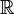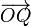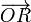# Vector (mathematics)

A vector is a widely used concept in mathematics, physics and all related sciences. Intuitively, it may be seen as a quantity which has both a magnitude and a direction. For example in elementary physics, velocity has both a magnitude and a direction, whereas speed is a scalar quantity with only a magnitude (it is the length of the velocity vector). A typical visualization of a 2- or 3-dimensional vector is an arrow. More generally, a vector can be described as a n-tuple of numbers that transforms in a specific way by transformations of the frame of reference.

In mathematics, an abstract concept of vector space has been introduced. It describes in an axiomatic way the detailed properties one expects of objects that can be labeled as 'vectors'. Thus, a vector is defined as a member of any vector space. Typical vector spaces include the real line, the Euclidean plane or space, the set of continuous functions on the line (with the supremum norm taken to be the length of a vector).

In general, vectors may be superimposed (linearly combined) to give new vectors. The most important property that makes a collection of vectors into a vector space is that superimposed vectors are again elements of the vector space (a vector space is closed under vector addition).

## Vector addition in the Euclidean plane

The prototype of a vector space is the Euclidean plane provided with an origin. The Euclidean plane, well-known from high-school geometry, is a collection of points (a 2-dimensional affine space) between which a distance is defined. One can single out a point O, the origin, and consider the collection of arrows with tail in O and heads in arbitrary points of the plane. Each arrow may be seen as a vector and all the arrows together form a two-dimensional vector space.

The most important characteristics of a vector space are the following:

1. It is defined with respect to a set of numbers (algebraically this set of numbers must constitute a field). We will consider the field of real numbers$\mathbb{R}$.
2. Vectors can be linearly combined (multiplied by elements of the underlying field and mutually added).

In Figure 1 these properties are illustrated. The addition of vectors (arrows) is by the parallelogram rule.

The length of the vector$\overrightarrow{OP}$ is equal to the distance between points O and P. Each vector can be multiplied by a real number, which scales the length of the vector. In Figure 1,$\overrightarrow{OP}$ is scaled by a factor 2,$\overrightarrow{OQ}$ by a factor 1.5. The scaled vectors have the same direction as the original vectors.

Two vectors can be added by drawing a parallelogram, exemplified by the dashed (blue) line segments in Figure 1. The dashed (blue) line segment P′–R is parallel to, and of the same length as, OQ′. This dashed segment is a parallel translation of OQ′ such that its tail coincides with P′. The other dashed line segment is a parallel translation of OP′ such that its tail coincides with Q′. The long diagonal of the parallelogram thus obtained is the vector$\overrightarrow{OR}$ resulting from the linear combination of$\overrightarrow{OP}$ and$\overrightarrow{OQ}$,$\overrightarrow{OR} = 2\;\overrightarrow{OP} + 1.5\; \overrightarrow{OQ}.$

The arrow of length 0 with tail and head in the origin O serves as the zero vector.